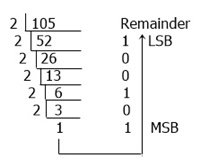# Decimal to Binary Conversion (with Fractional Part)

In this article, we will learn how to convert a number from the decimal system to the binary system, and we will also look at an example. But before we get started, let's make sure we're clear on what these two numbers represent.

• Decimal number: Decimal numbers are those that are made up of the digits 0, 1, 2, 3, 4, 5, 6, 7, 8, and 9. For example: 1203, 3209, 80, etc.
One more thing regarding decimal numbers that you need to keep in mind is that they have a base of 10, as shown here. When a number is expressed using the base 10 system, it has a total of 10 digits to represent it. These ten digits range from zero to nine.
• Binary number: On the other hand, binary numbers have a base of 2, which indicates that a binary number is comprised of a total of two digits, which are the digits 0 and 1. For example, 1101, 111, and 100011, etc.

## Decimal to Binary Conversion with an Example

Any decimal number can be converted into a binary number by dividing the decimal number by the base of the binary number system, which is 2. Let's look at an example of this conversion's working rule so that we can get a better understanding of it.

Now that we have a number in the decimal number system, let's say 105, our task is to find that number or one that is equivalent to it in binary form or in the binary number system. Let's focus on the information presented in the following figure:From the above figure, we will get 1101001, which is the same number as 105, but in the binary number system. Therefore, (105)10 = (1101001)2.

## Decimal (containing a fractional part) to Binary Conversion Steps

Here are the steps or rules of multiplication for fractional part conversion:

• Multiply the given fractional decimal number by the base r (2 here).
• Now record the carry generated in this multiplication as HSB.
• Only multiply the fractional number of the product in step 2 by the base 2, and save the carry as the next bit to MSB.

Let’s take an example to apply the above steps practically and learn how we can convert any fractional part value given in the decimal number system to the binary version of the same fractional part.

In this case,.42 must be converted to binary. That is, (.42)10 = ( ? )2.

The table given below shows the step-by-step multiplication of the fractional part as directed in the above rules.

Decimal Fractional Multiply with Base Multiplication Result Carry Value
.42 * 2 0.84 0 MSB
.84 * 2 1.68 1
.68 * 2 1.36 1
.36 * 2 0.72 0
.72 * 2 1.44 1 LSB

As you can see, the multiplication procedure

• First we have to multiply the complete fractional part, that is .42 with base equals 2
• Then we have the product of 0.84, and in this number we have the carry record of 0. Because we must take the number present before the decimal point, 0 is the carry record here.
• And when we multiply.84 by 2, we get 1.68 as a multiplication result, and we can see that the carry is 1.
• Similarly, in third, fourth, and fifth multiplication, we have carry as 1, 0, and 1.
• Now, after combining all the carry records, we will get 01101 as a result.

As a result, this is equivalent to .42, which is 0.01101. Or, (0.42)10 = (0.01101)2.

#### Programs Created on Decimal to Binary Conversion

Computer Fundamentals Quiz

« Previous Tutorial Next Tutorial »